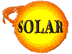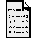Is the Sun Shrinking?(by Amara Graps)

There have been claims over the years that the Sun is contracting slowly over time. Here, we examine that claim.

Let us assume that the Sun is shrinking is by gravity. Then from the equation that scientists have for the change of the Sun's luminosity (luminosity is an energy output) versus its radius, the Sun would be shrinking in its radius 74 centimeters per year. We would have detected such a noticeable change over the past history (over 500 years this would be a 0.005 arc seconds difference in the radius of the Sun from our viewing position on the Earth), and we haven't detected such a change. So our observations don't show the Sun to be shrinking by gravitational contraction.

What about the Sun's mass becoming less by its process of producing energy (fusion)?

The Sun actually does lose mass in the process of producing energy. Let us see how much.

We can use the following numbers from Kenneth R. Lang's book: _Astrophysical Data_:

```Solar Mass = 1.989 x 1033 g
Absolute luminosity = 3.86 x 1033 erg/sec
Speed of light c   = 2.99 x 1010 cm/sec
```

Start with Einstein's famous equation: "E = mass times c2" and rearrange the terms to solve for the mass M:

M = E/c2

And after inputting our numbers:

```        = 3.86x1033/(2.99x1010)2
= 4.289x1012 g/sec
```
we find that the Sun loses mass 4.289x1012 g every second to energy. Or, in other units, the Sun loses mass 1.353x1020 g every year to energy.

The Sun is thought to have a remaining lifetime of about 5x109 years. If we assume that the Sun's rate of fuel consumption (the luminosity value given above) remains constant (it won't, but it isn't a bad assumption) in the remaining time of 5x109 years, then let us see how much mass the Sun will convert to energy in its remaining lifetime.

Mass = (1.353x1020 g/year) * 5x109 years = 6.8 x 1029 g

In units of tons, every second, the Sun's fusion processes are converting about 700 million tons of hydrogen into helium "ashes". In doing so, 0.7 percent of the hydrogen matter (5 million tons) disappears as pure energy. (My reference for this paragraph is "The Sun" chapter in _The New Solar System_ editor: Beatty and Chaikin, Sky Publishing Press.)

Since the Sun's current mass is 1.989 x 1033 g, the percentage of its current mass that will be converted to energy is:

6.8 x 1029 g / 1.989 x 1033 g = 0.00034 of its current mass or .034 percent.

In other words, the Sun's mass at the end of its lifetime is 99.966% of its current mass. See.. nothing to worry about!

Note that our Solar System is a very active place. Comets fall into the Sun often (the SOHO spacecraft has detected many these "sun grazing and sun-colliding comets"). And dust generated by asteroids hitting each other and comets coming into the inner Solar System and releasing dust, creates tons of very fine dust particles that fall into the Sun every second. So you see, the Sun doesn't always "lose."

In 1987, several astronomers from Paris Observatory made an announcement regarding the size of the Sun that astonished their colleagues (Kippenhahn, R., 1994, pg. 163). They claimed that solar eclipse data from 1666 to 1719 showed that the Sun was 2000 kilometers larger than it is today. This amounts to a 0.3 percent reduction; and the time period, which roughly corresponds to the Maunder Minimum, seemed to be more than coincidental. However, this data was found to contain an error regarding the 1715 solar eclipse path of totality. Consequently, the Sun was the same size in 1716 as it is today, and astronomers were reassured.

#### Reference

R. Kippenhahn, Discovering the Secrets of the Sun, Wiley Press, 1994.

Back to the FAQsBack to the SOLAR CenterComments?

Special Thanks to A. Graps